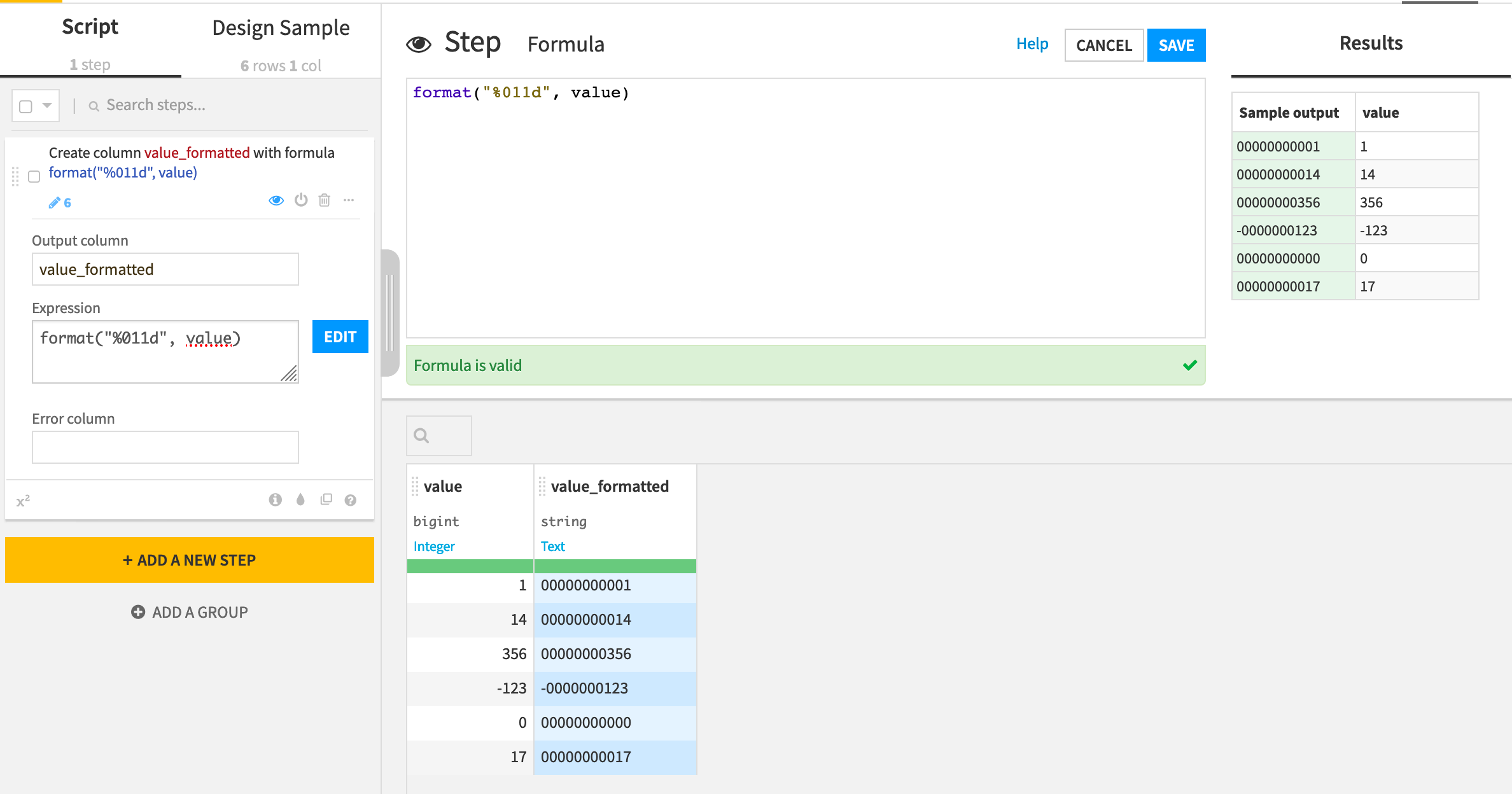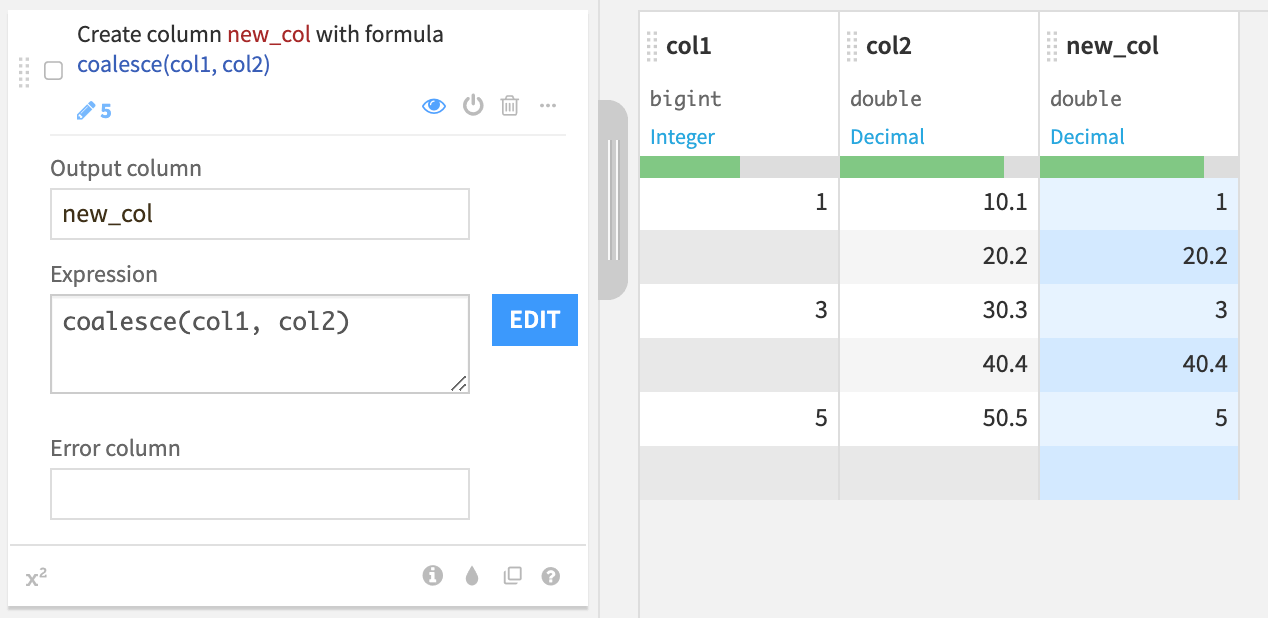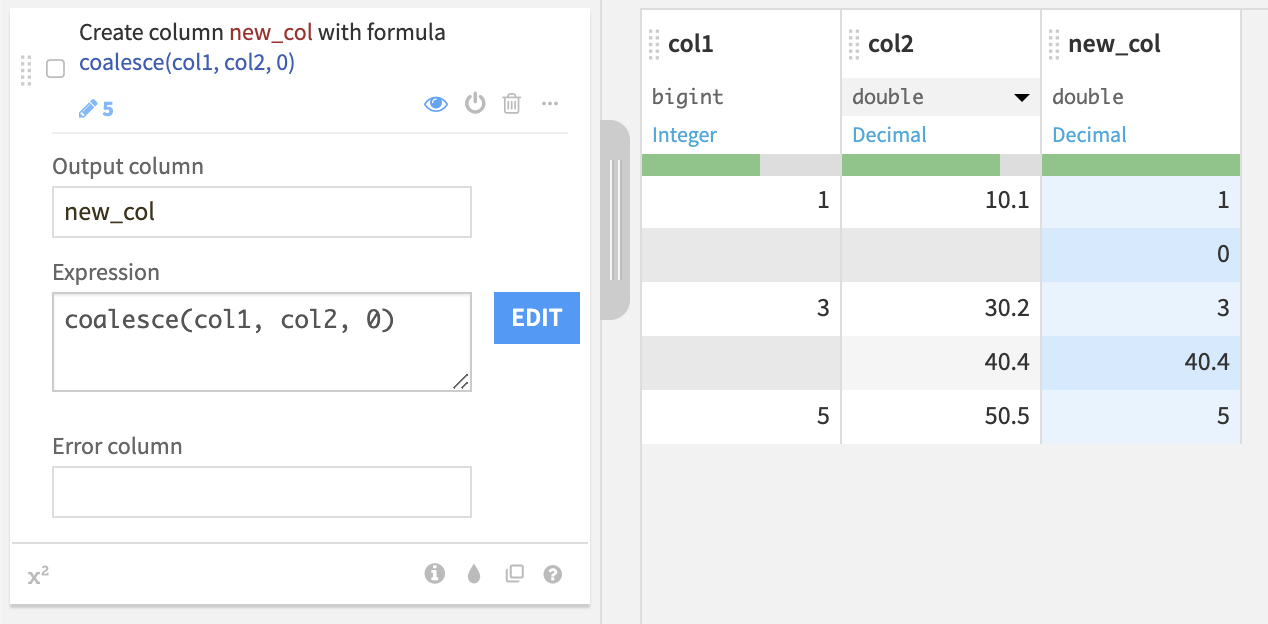# Dataiku Formulas¶

Explore resources for working with Dataiku formulas, which can be applied in the Prepare recipe, as well as many other locations.

## How-to | Remove scientific notation in a column¶

Note

In version 11.1 and above, in columns of type Decimal, numbers below 1015 (i.e. up to 999,999,999,999,999) are automatically formatted using the decimal notation in the dataset Explore view. Similarly, numbers above 10-15 (i.e. down to 0.000000000000002) are now automatically formatted using the decimal notation. In version 11.0 and earlier, the thresholds were 107 and 10-7.

You can also switch scientific notation on or off for numbers between 10-7 and 10-15 and between 107 and 1015 by selecting Toggle scientific notation from the column name dropdown in a Prepare recipe or dataset Explore view.

Formatting numbers can often be a tedious data cleaning task.

It can be made easier with the format() function of the Dataiku Formula language. This function takes a printf format string and applies it to any value.

Format strings are immensely powerful, as they allow you to truncate strings, change precision, switch between numerical notations, left-pad strings, pad numbers with zeros, etc. More specifically, Dataiku formulas use the Java variant of format strings.

For example, you may have a column of very small numbers represented in scientific notation. If instead you wanted to convert this column to 5 decimal places, you can use:

format("%.5f", my_column_name)


Note

For the change in format to persist in the output of the Prepare recipe, you must change the storage type of the formatted column to a string. You can do this from the storage type dropdown of the column header, while in the recipe editor.

For a deeper explanation of this behavior, please see the reference documentation on variable typing and auto-typing in the formula language.

### What’s Next?¶

A common requirement when you have a column of numbers is to format all numbers so that they have the same length, adding leading zeros if needed.

This can be done in the Prepare recipe using a Formula.

The formula function to use is format. For example, to ensure that all values of the column mycolumn are padded to have a length of 11, including leading zeros, use: format("%011d", mycolumn)It is useful to remember the usual formula rules to refer the values of columns, as described in the Dataiku reference documentation.

For example, to do the same in a column named my column (note the space), you would use instead format("%011d", numval("my column"))

format is actually a very powerful function that uses Java string formatting capabilities. See this Java reference for more details.

## How-to | Fill empty cells of a column with the value of the corresponding row from another column¶

Handling missing data is one data preparation challenge that analysts routinely face. Should you discard observations with missing values or perhaps impute missing values with a summary value like the median?

To handle missing data, the Prepare recipe has dozens of built-in processors ready to solve many of the most common challenges without any coding. In addition, Dataiku has its own Formula language to craft more custom solutions.

For example, in some cases, you may want to fill the empty cells of a column with values of the corresponding rows from another column.

In a Prepare recipe, use the Formula processor with the coalesce() function as shown below:Here we fill the empty values of col1 with the corresponding values of col2 in a new column.

You can also specify multiple columns, or even directly specify the missing values.Here we fill the empty values of col1 with the values of col2, or 0 when col2 is also empty.

The Formula language gives you the flexibility to achieve more customized tasks. For example, you can combine functions in the same expression.Here we fill the empty values of col1 with the corresponding floored values of col2 in a new column.

## FAQ | In a formula, how to check if a variable belongs to a set of values?¶

A common need when using Formulas is to check whether a variable (generally, a column) belongs to a set of values.

For example, you may want to check if mycolumn has value 1, 2 or 3. To do that in a formula, use the arrayContains function:

arrayContains([1,2,3], mycolumn)


In other words, instead of checking if “mycolumn is in [1,2,3]”, you check if “[1,2,3] contains mycolumn”.

It may be useful to recall the reference documentation on reading column values.

For example, to do the same in a column named my column (note the space), you would use:

arrayContains(["a", "b", "c"], strval("my column"))


If you wanted to replace specific values with a variable, normal variable syntax rules apply.

arrayContains([${my_var1},${my_var2}, 3], mycolumn)